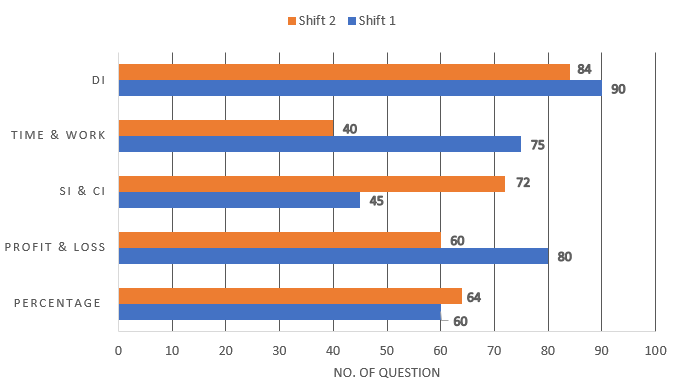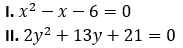Dear Aspirants,

Quantitative Aptitude Quiz For SBI PO/Clerk Prelims

Numerical Ability or Quantitative Aptitude Section has given heebie-jeebies to the aspirants when they appear for a banking examination. As the level of every other section is only getting complex and convoluted, there is no doubt that this section, too, makes your blood run cold. The questions asked in this section are calculative and very time-consuming. But once dealt with proper strategy, speed, and accuracy, this section can get you the maximum marks in the examination. Following is the Quantitative Aptitude quiz to help you practice with the best of latest pattern questions.

Directions (1-5): Study the following bar graph carefully and answer the following questions.
The bar graph shows the no. of questions come from different sections of quantitative aptitude in two shifts of SBI clerk prelims exam.Q1. No. of questions asked in shift 1 from SI & CI is what percent of no. of questions asked from time and work in the same shift?
60%
50%
55%
61%
75%

Q2. Find the average no. of questions asked from all the given sections in shift 2.
46
64
48
68
74

Q3. What is the ratio of no. of questions asked from profit & loss and percentage together in shift 1 to the questions asked from profit & loss and time & work together in shift-2?
6 : 5
5 : 7
7 : 5
5 : 6
8 : 7

Q4. What is the difference between total no. of questions asked in both shifts from all the given sections together?
45
40
35
30
60

Q5. Total no. of questions asked from DI in shift 2 are how much percent less than that in shift 1?
5⅔%
6⅔%
4⅔%
8%
3⅔%

Directions (6-10): The following table shows the total no. of candidates who has cleared the SBI Clark prelims exam 2018 and ratio of male to female in them from five different states of India. Study the table carefully to answer the following questions.Q6. Find the total no. of male candidates who qualified the SBI clerk prelims exam from UP and Maharashtra together.
16400
17900
15900
21400
18600

Q7. Find the difference between total male candidates from Delhi and Bihar together to the total female candidates from same states together?
3600
3200
2800
2400
3800

Q8. Total male candidate of Gujrat are what percent of total male candidates of Maharashtra?

Q9. What is the average no. of candidates who cleared SBI Clark Pre- exam from all states together?
9560
10450
1560
11560
8650

Q10. Female candidates of UP are what percent more or less than that from Bihar?

Directions (11-15): Two equations I and II are given below in each question. You have to solve these equations and give answer

Q11.if x < y
if x > y
if x ≤ y
if x ≥ y
if x = y or no relation can be established

Q12.if x < y
if x > y
if x ≤ y
if x ≥ y
if x = y or no relation can be established

Q13.if x < y
if x > y
if x ≤ y
if x ≥ y
if x = y or no relation can be established

Q14.if x < y
if x > y
if x ≤ y
if x ≥ y
if x = y or no relation can be established

Q15.if x < y
if x > y
if x ≤ y
if x ≥ y
if x = y or no relation can be established

You May also like to Read: